# Tetration Forum

Full Version: Integration?
You're currently viewing a stripped down version of our content. View the full version with proper formatting.
Pages: 1 2
Hello!

I tried integrating the function x^^n for any nonnegative integer n. I wanted to share my method and results, so that you can check fofr accuracy (if I've done it correctly, it'd be a rare occurance- I haven't taken calculus as a class yet, so I went off of what I've read in books).

First we write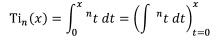Then we can expand on the indefinite integral in the parentheses.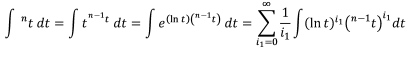Once we have calculated this out, we can use substitution.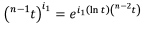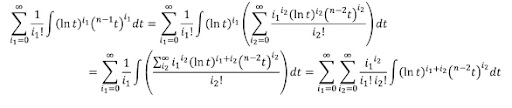If we repeat this process n-1 times, then we get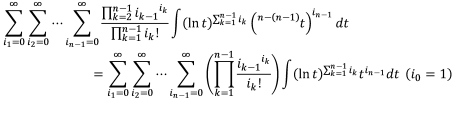Notice that I defined i_0 = 1. This merely simplifies the notation.
I haven't yet completed that general integral, but what matters is that it can be completed on a case-by-case basis through integration by parts. This is demonstrated for n=2...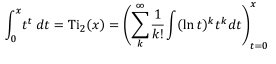Integrating by parts,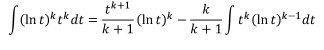If we do this indefinitely, we end up with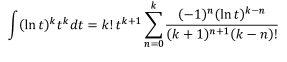Plugging in,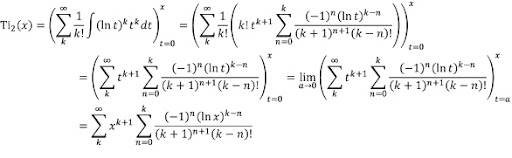Bernoulli's integral, the so-called "Sophomore's Dream", is calculated as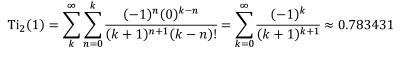Note that 0^0 is 1, so the second summation only has a non-zero value when n=k.

If anyone has any corrections, or if this has been done before, I'd love to know.Also, it'd be cool to see graphs of these functions from anyone who has Maple or Mathematica (or other amazing program).

Edit: Ugh. Just noticed that in the last few equations, I wrote the summations wrong. Rest assured, it's from k=0 to infinity.
Nice done.
So you reduce the integral to a series containing x and ln(x).
Another way to do it would be to just integrate the Taylor expansion of at 1. (See also this thread.)
Unfortunately, I have no idea what the Taylor series is. What you linked to uses x and ln(x), while a true Taylor series would only involve x. It's be cool to see how to calculate the nth derivative of , because then one could use the Taylor series to find the Taylor series of the integral, which would probably be simpler to calculate than my solution.

On another note, I decided to tackle . Here, stands for . Integration by parts yielded . Thus,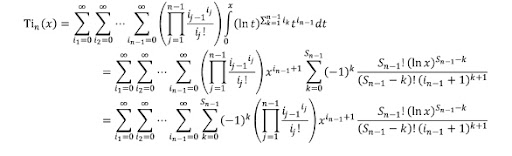which I'm not going to bother TEXing.

Unfortunately, all those summations make calculating even extraordinarily difficult. Can anyone calculate this series with Maple or Mathematica? (I know that the integral approximation is ~0.573... but I want to check my series with that)
(07/02/2010, 09:35 PM)73939 Wrote: [ -> ]Unfortunately, I have no idea what the Taylor series is. What you linked to uses x and ln(x), while a true Taylor series would only involve x.

(07/02/2010, 09:35 PM)73939 Wrote: [ -> ]Unfortunately, all those summations make calculating even extraordinarily difficult. Can anyone calculate this series with Maple or Mathematica? (I know that the integral approximation is ~0.573... but I want to check my series with that)

I use sage with my formal_powerseries package. With a code like this
Code:
sage: load formal_powerseries.py sage: F = FormalPowerSeriesRing(QQ) sage: pt3 = F.Exp(F.Log_inc * F.Exp(F.Log_inc * F.Inc)) sage: pt3 [1, 1, 1, 3/2, 4/3, 3/2, 53/40, 233/180, 5627/5040, 2501/2520, 8399/10080, ...] sage: #or the derivatives sage: pt3.mul_fact() [1, 1, 2, 9, 32, 180, 954, 6524, 45016, 360144, 3023640, 27617832, ...]

we get the Taylor coefficients of at 0 which is the same as the Taylor coefficients of at 1.

We can now integrate this:
Code:
sage: pt3i = pt3.integral() sage: pt3i [0, 1, 1/2, 1/3, 3/8, 4/15, 1/4, 53/280, 233/1440, 5627/45360, 2501/25200, ...] sage: pt3i.mul_fact() [0, 1, 1, 2, 9, 32, 180, 954, 6524, 45016, 360144, 3023640, 27617832, ...]

I always include the derivatives which is the powerseries coefficients multiplied by n! because this is an integer sequence, which you possibly can look up at Sloane's Online Encypclopedia of Integer Sequences. And now I see that the sequence for the 3rd powertower (or its integral which is just the series shifted to the right by one) isnt found there (the second powertower is of course contained). I will filed a new submission.

And now we can compute the integral pt3i(1-1) - pt3i(0-1) = -pt3i(-1) by truncating the infinite series, say to 100. To have an idea about the accuracy we also calculate the 101-truncation:

Code:
sage: p = pt3i.polynomial(100) sage: p2 = pt3i.polynomial(101) sage: RR(-p(-1)) 0.573116317784245 sage: RR(-p2(-1)) 0.573116465191639

This means .

Edit: If we improve precision a bit taking a polynomial with 200 terms which takes a bit longer to compute we see that the last digits are not accurate:
Code:
sage: p3 = pt3i.polynomial(200) sage: p3x = pt3i.polynomial(201) sage: RR(-p3(-1)) 0.573120826997061 sage: RR(-p3x(-1)) 0.573120837278469

This time a bit more careful with the last digits I would say: .

Caution: actually one need to be careful with this kind of evaluation of a powerseries. Because we know that the convergence radius of the powertowers are at most 1. That means that -1 is at boundary of the disk of convergence of pt3, so it may or may not converge. And I guess the same is true for pt3i.
(07/03/2010, 11:34 AM)bo198214 Wrote: [ -> ][quote='73939' pid='4939' dateline='1278102954']
(...)
This time a bit more careful with the last digits I would say: .

Pari/GP gives using numerical integration and internal float-precision of 200 digits

gp> intnum(x=-1,0,(1+x)^(1+x)^(1+x))
%1 = 0.573121567043619582758830

[update] I also had tried it with my matrix-formulation and my simple possibilities of convergence-acceleration using Euler- et al summation.
Having the same taylor-series with which Henryk worked, an improvement would only be possible, if such a convergence-acceleration were effective. But what I see if I look at the terms of the powerseries of the integral with the powers of x(=0 and =-1) mutiplied in, is that the summands decrease slowly to zero (?) but not with alternating sign - so common convergence-accelerators should not work easily. (The extreme case would be to try to sum the harmonic series, where such convergence acceleration would even be contradictory).
So with all my usual tools I come not nearer than four to six digits (using 64 terms) like Henryk, - thus, to apply this model of powerseries at all, would require some more consideration before ...
[/update]
Gottfried
I have also worked out an integral for x^x. Mine involves the incomplete gamma function.
That's pretty interesting. I'd certainly like to see that. Could you post it?
On another note concerning integration:
Could the function be defined as some integral ? The Gamma function and the Beta function, both extending some function on the integers (factorial and binomial coefficients, respectively), are defined in such a way, so it stands to reason there might be a similar integral for tetration.
It may be useful that

In other words,

Pages: 1 2